## Algebra

Showing posts with label complex fraction. Show all posts
Showing posts with label complex fraction. Show all posts

### Elementary Algebra Exam #4

Click on the 10 question exam covering topics in chapter 7 (Rational Expressions and Equations). Give yourself one hour to try all of the problems and then come back and check your answers.

Simplify (Assume all denominators are nonzero.)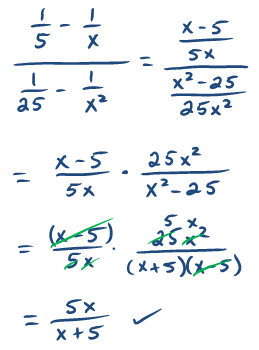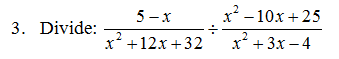Perform the operations and state the restrictions.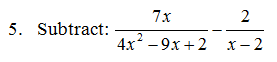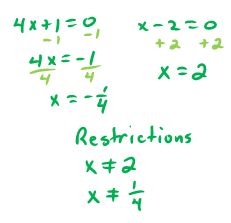Solve.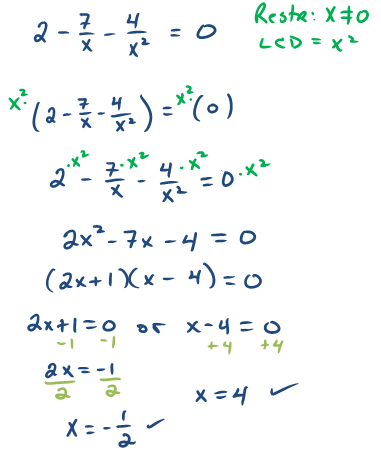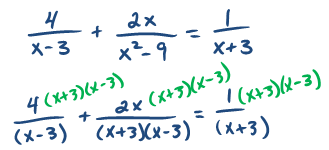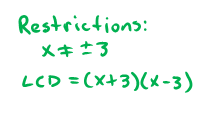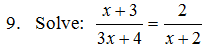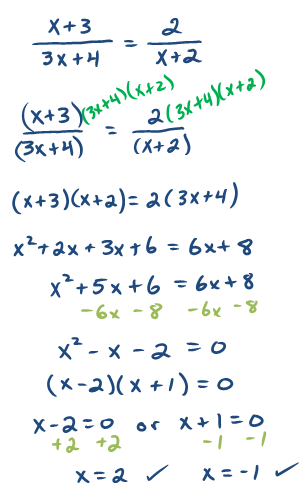10. The sum of the reciprocals of two consecutive odd integers is 4/3.  Set up an algebraic equation and use it to find the two integers.---